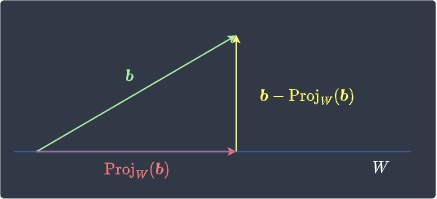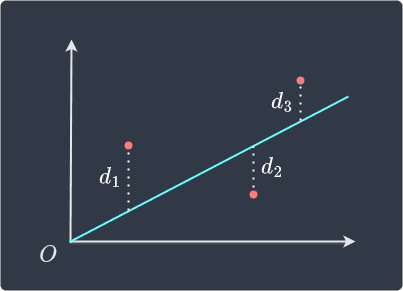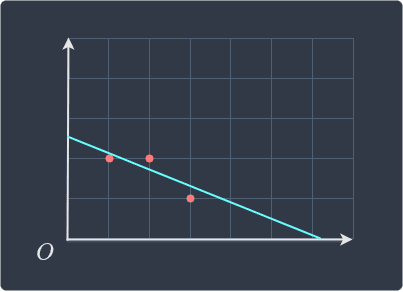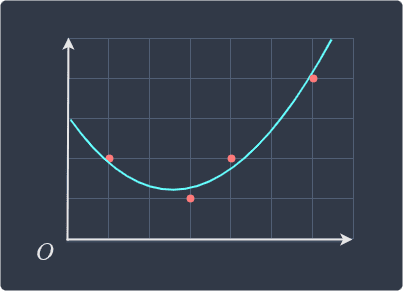search
Search
Unlock 100+ guides
search toc
close
account_circle
Profile
exit_to_app
Sign out
search
keyboard_voice
close
Searching Tips
Search for a recipe:
"Creating a table in MySQL"
Search for an API documentation: "@append"
Search for code: "!dataframe"
Apply a tag filter: "#python"
Useful Shortcuts
/ to open search panel
Esc to close search panel
to navigate between search results
d to clear all current filters
Enter to expand content previewDoc SearchCode Search BetaSORRY NOTHING FOUND!
mic
Start speaking...Voice search is only supported in Safari and Chrome.
Shrink
Navigate to

# Guide on Least squares in Linear Algebra

schedule Aug 12, 2023
Last updated
local_offer
Linear Algebra
Tags
mode_heat
Master the mathematics behind data science with 100+ top-tier guides
Start your free 7-days trial now!

# Motivation

Consider the system $\boldsymbol{Ax}=\boldsymbol{b}$ where:

$$\boldsymbol{A}=\begin{pmatrix} 1&0\\1&2\\0&1 \end{pmatrix},\;\;\;\;\; \boldsymbol{b}= \begin{pmatrix} 1\\2\\2 \end{pmatrix}$$

In matrix form, the system is:

$$\begin{equation}\label{eq:Stoc8pzDDFCy4udlqXj} \begin{pmatrix} 1&0\\1&2\\0&1 \end{pmatrix} \begin{pmatrix} x_1\\x_2 \end{pmatrix}= \begin{pmatrix} 1\\2\\2 \end{pmatrix} \end{equation}$$

Using theoremlink, the left-hand side can be written as:

$$\begin{pmatrix} 1\\1\\0 \end{pmatrix}x_1+ \begin{pmatrix} 0\\2\\1 \end{pmatrix}x_2= \begin{pmatrix} 1\\2\\2 \end{pmatrix}$$

We can see that the system has no solution, that is, the system is inconsistentlink. Instead, we can compromise and find values for $x_1$ and $x_2$ such that we approximately get $\boldsymbol{b}$. For instance, consider the following candidate:

$$\boldsymbol{x}_{(1)}= \begin{pmatrix} 10\\20 \end{pmatrix}$$

This candidate yields:

$$\begin{equation}\label{eq:tz86cAg7gxXTITJtXAf} \begin{pmatrix} 1\\1\\0 \end{pmatrix}(10)+ \begin{pmatrix} 0\\2\\1 \end{pmatrix}(20)= \begin{pmatrix} 10\\50\\20 \end{pmatrix} \end{equation}$$

Intuitively, we know that $\boldsymbol{x}_{(1)}$ is bad because the resulting vector is very different from $\boldsymbol{b}$.

Now, let's consider another candidate:

$$\boldsymbol{x}_{(2)}= \begin{pmatrix} 1\\2 \end{pmatrix}$$

This candidate yields:

$$\begin{equation}\label{eq:Dl6E0pbQEuANwC3LOue} \begin{pmatrix} 1\\1\\0 \end{pmatrix}(1)+ \begin{pmatrix} 0\\2\\1 \end{pmatrix}(2)= \begin{pmatrix} 1\\5\\2 \end{pmatrix} \end{equation}$$

We see that $\boldsymbol{x}_{(2)}$ is much better than $\boldsymbol{x}_{(1)}$ because the resulting vector is much closer to $\boldsymbol{b}$. To numerically compare which candidate is better, we can compute the distance between the resulting vector $\boldsymbol{Ax}$ and the true vector $\boldsymbol{b}$ like so:

$$\Vert\boldsymbol{A}\boldsymbol{x}-\boldsymbol{b}\Vert$$

For instance, the performance of $\boldsymbol{x}_{(1)}$ is:

\begin{align*} \Vert\boldsymbol{A}\boldsymbol{x}_{(1)}-\boldsymbol{b}\Vert &= \left\Vert\begin{pmatrix}1&0\\1&2\\0&1\end{pmatrix} \begin{pmatrix}10\\20\end{pmatrix}-\begin{pmatrix}1\\2\\2\end{pmatrix}\right\Vert\\ &=\left\Vert\begin{pmatrix}9\\48\\18\end{pmatrix}\right\Vert\\ &=\sqrt{9^2+48^2+18^2}\\ &\approx52 \end{align*}

Similarly, the performance of $\boldsymbol{x}_{(2)}$ is:

\begin{align*} \Vert\boldsymbol{A}\boldsymbol{x}_{(2)}-\boldsymbol{b}\Vert&=3 \end{align*}

We can see that the performance of $\boldsymbol{x}_{(2)}$ is indeed much better than $\boldsymbol{x}_{(1)}$. The key question is whether or not there exist even better candidates that produce a vector closer to $\boldsymbol{b}$. This is what is known as the least squares problem, which we formally state below.

Definition.

# Least squares problem

Consider an inconsistent linear system $\boldsymbol{Ax}=\boldsymbol{b}$ where $\boldsymbol{A}$ is an $m\times{n}$ matrix and $\boldsymbol{x},\boldsymbol{b}\in\mathbb{R}^n$. The objective is to find $\boldsymbol{x}$ such that the distance between $\boldsymbol{b}$ and $\boldsymbol{Ax}$ is minimized, that is:

$$\min \Vert{\boldsymbol{b}-\boldsymbol{Ax}}\Vert$$

The particular $\boldsymbol{x}$ that minimizes $\Vert\boldsymbol{b}-\boldsymbol{Ax}\Vert$ is called the least squares solution of $\boldsymbol{Ax}=\boldsymbol{b}$.

Theorem.

# Best approximation theorem

Let $W$ be a finite-dimensional subspace of a vector space $V$. If $\boldsymbol{b}$ is in $V$ and $\boldsymbol{w}$ is any vector in $W$, then $\mathrm{Proj}_W(b)$ is the best approximation of $\boldsymbol{b}$, that is:

$$\Big\Vert\boldsymbol{b}-\boldsymbol{w}\Big\Vert\gt \Big\Vert\boldsymbol{b}-\mathrm{Proj}_W(\boldsymbol{b})\Big\Vert$$

This means that the distance between $\boldsymbol{b}$ and $\mathrm{Proj}_W(\boldsymbol{b})$ is shorter than the distance between $\boldsymbol{b}$ and any other vector $\boldsymbol{w}$ in $W$.

Proof. Let $\boldsymbol{w}$ be any vector in $\boldsymbol{W}$ and let $\boldsymbol{b}$ be a vector in $V$. The vector $\boldsymbol{b}-\boldsymbol{w}$ can be written as:

$$\begin{equation}\label{eq:NtPBCQvv0XvU0cwg2yW} \boldsymbol{b}-\boldsymbol{w}= \big(\boldsymbol{b}-\mathrm{Proj}_W(\boldsymbol{b})\big)+ \big(\mathrm{Proj}_W(\boldsymbol{b})-\boldsymbol{w}\big) \end{equation}$$

Visually, the first term on the right-hand side of \eqref{eq:NtPBCQvv0XvU0cwg2yW} is:We see that $\boldsymbol{b}-\mathrm{Proj}_W(\boldsymbol{b})$ is orthogonal to vectors on $W$. This means that $\boldsymbol{b}-\mathrm{Proj}_W(\boldsymbol{b})$ belongs to the orthogonal complementlink of $W$, that is:

$$\big(\boldsymbol{b}-\mathrm{Proj}_W(\boldsymbol{b})\big) \in{W^\perp}$$

The vector $\mathrm{Proj}_W(\boldsymbol{b})$ represents the projected vector of $\boldsymbol{b}$ onto $W$, which means that $\mathrm{Proj}_W(\boldsymbol{b})\in{W}$. Clearly, $\boldsymbol{w}\in{W}$ as well. Because $W$ is a subspacelink, adding any two vectors in $W$ results in another vector in $W$. Therefore, we have that:

$$\big(\mathrm{Proj}_W(\boldsymbol{b})-\boldsymbol{w}\big)\in{W}$$

Because the two vectors on the right-hand side of \eqref{eq:NtPBCQvv0XvU0cwg2yW} are orthogonal, we can apply the Pythagoras theorem to get:

$$\begin{equation}\label{eq:M5Q0KC5padiBg2sdZiB} \Big\Vert\boldsymbol{b}-\boldsymbol{w}\Big\Vert^2= \Big\Vert\boldsymbol{b}-\mathrm{Proj}_W(\boldsymbol{b})\Big\Vert^2+ {\color{green}\Big\Vert\mathrm{Proj}_W(\boldsymbol{b})-\boldsymbol{w}\Big\Vert^2} \end{equation}$$

Every term in \eqref{eq:M5Q0KC5padiBg2sdZiB} is a magnitude and is therefore non-negative. Removing the green term will give us the following inequality:

$$\Big\Vert\boldsymbol{b}-\boldsymbol{w}\Big\Vert^2\gt \Big\Vert\boldsymbol{b}-\mathrm{Proj}_W(\boldsymbol{b})\Big\Vert^2$$

Taking the square root of both sides gives:

$$\Big\Vert\boldsymbol{b}-\boldsymbol{w}\Big\Vert\gt \Big\Vert\boldsymbol{b}-\mathrm{Proj}_W(\boldsymbol{b})\Big\Vert$$

Note that we don't have to care about the sign because magnitudes are non-negative. This completes the proof.

Theorem.

# Normal equation

The set of least squares solution of $\boldsymbol{Ax}=\boldsymbol{b}$ is given by the so-called normal equation:

$$\begin{equation}\label{eq:cwjz0UCZN7VxKmAF1uy} \boldsymbol{A}^T\boldsymbol{Ax} =\boldsymbol{A}^T\boldsymbol{b} \end{equation}$$

Proof. Let $W$ be the column spacelink of $\boldsymbol{A}$, that is, $W=\mathrm{col}(\boldsymbol{A})$. Since $\mathrm{Proj}_W(\boldsymbol{b})\in{W}$, there exists some $\boldsymbol{x}$ such that:

$$\boldsymbol{Ax}=\mathrm{Proj}_W(\boldsymbol{b})$$

Let's subtract both sides from $\boldsymbol{b}$ to get:

$$\boldsymbol{b}-\boldsymbol{Ax}= \boldsymbol{b}-\mathrm{Proj}_W(\boldsymbol{b})$$

Multiplying both sides by $\boldsymbol{A}^T$ yields:

$$\begin{equation}\label{eq:AX0WcTyXjuTZP1bq5q3} \boldsymbol{A}^T(\boldsymbol{b}-\boldsymbol{Ax})= \boldsymbol{A}^T\big( \boldsymbol{b}-\mathrm{Proj}_W(\boldsymbol{b})\big) \end{equation}$$

The relationship between $\boldsymbol{b}$ and $\mathrm{Proj}_W(\boldsymbol{b})$ is visualized below:The vector $\boldsymbol{b}-\mathrm{Proj}_W(\boldsymbol{b})$ is orthogonal to $W$, which means that:

$$\boldsymbol{b}-\mathrm{Proj}_W(\boldsymbol{b}) \in W^\perp$$

Since the subspace $W$ is equal to the column space of $\boldsymbol{A}$, we get:

$$\begin{equation}\label{eq:LWnxqVcatgLzsv2aLad} \boldsymbol{b}-\mathrm{Proj}_W(\boldsymbol{b}) \in \big(\mathrm{col}(\boldsymbol{A})\big)^\perp \end{equation}$$

$$\mathrm{nullspace}(\boldsymbol{A}^T) =(\mathrm{col}(\boldsymbol{A}))^\perp$$

$$\boldsymbol{b}-\mathrm{Proj}_W(\boldsymbol{b}) \in \mathrm{nullspace}(\boldsymbol{A}^T)$$

By definitionlink of null space, we have that:

$$\begin{equation}\label{eq:CHxLJWjQkgtuphSRuSJ} \boldsymbol{A}^T \big(\boldsymbol{b}-\mathrm{Proj}_W(\boldsymbol{b})\big) =\boldsymbol{0} \end{equation}$$

Substituting \eqref{eq:CHxLJWjQkgtuphSRuSJ} into the right-hand side of \eqref{eq:AX0WcTyXjuTZP1bq5q3} gives:

$$\boldsymbol{A}^T(\boldsymbol{b}-\boldsymbol{Ax})= \boldsymbol{0}$$

Rearranging gives:

\begin{align*} \boldsymbol{A}^T(\boldsymbol{b}-\boldsymbol{Ax}) &=\boldsymbol{0}\\ \boldsymbol{A}^T\boldsymbol{b}- \boldsymbol{A}^T\boldsymbol{Ax} &=\boldsymbol{0}\\ \boldsymbol{A}^T\boldsymbol{Ax} &=\boldsymbol{A}^T\boldsymbol{b}\\ \end{align*}

This completes the proof.

Example.

# Finding the least squares solution

Consider the system $\boldsymbol{Ax}=\boldsymbol{b}$ where:

$$\boldsymbol{A}=\begin{pmatrix} 1&0\\1&0\\0&1 \end{pmatrix},\;\;\;\;\; \boldsymbol{b}= \begin{pmatrix} 1\\2\\2 \end{pmatrix}$$

Find the least squares solution.

Solution. Let's first check whether we can solve the system exactly:

$$\begin{pmatrix} 1&0\\1&0\\0&1 \end{pmatrix} \begin{pmatrix} x_1\\x_2 \end{pmatrix}= \begin{pmatrix} 1\\2\\2 \end{pmatrix} \;\;\;\;\;\;\;\;\Longleftrightarrow\;\;\;\;\;\;\;\; \begin{pmatrix} 1\\1\\0 \end{pmatrix}x_1+ \begin{pmatrix} 0\\0\\1 \end{pmatrix}x_2= \begin{pmatrix} 1\\2\\2 \end{pmatrix}$$

Clearly, we cannot solve the system directly. The best we can do is to solve for the least squares solution that best approximates $\boldsymbol{b}$.

We know from theoremlink that the least squares solution $\boldsymbol{x}$ is given by:

$$\begin{equation}\label{eq:iI4IBl5CtvG0NSoxhfB} \boldsymbol{A}^T\boldsymbol{Ax} =\boldsymbol{A}^T\boldsymbol{b} \end{equation}$$

Firstly, $\boldsymbol{A}^T\boldsymbol{A}$ is:

$$\boldsymbol{A}^T\boldsymbol{A}= \begin{pmatrix}1&1&0\\0&0&1\end{pmatrix} \begin{pmatrix}1&0\\1&0\\0&1\end{pmatrix} = \begin{pmatrix}2&0\\0&1\end{pmatrix}$$

Next, $\boldsymbol{A}^T\boldsymbol{b}$ is:

$$\boldsymbol{A}^T\boldsymbol{b}= \begin{pmatrix}1&1&0\\0&0&1\end{pmatrix} \begin{pmatrix}1\\2\\2\end{pmatrix} = \begin{pmatrix}3\\2\end{pmatrix}$$

Therefore, \eqref{eq:iI4IBl5CtvG0NSoxhfB} is:

$$\begin{pmatrix}2&0\\0&1\end{pmatrix} \begin{pmatrix}x_1\\x_2\end{pmatrix} = \begin{pmatrix}3\\2\end{pmatrix}$$

We have that $x_1=1.5$ and $x_2=2$. Therefore, the least squares solution is:

$$\begin{pmatrix}x_1\\x_2\end{pmatrix} = \begin{pmatrix}1.5\\2\end{pmatrix}$$

Let's see how well this least squares solution approximates $\boldsymbol{b}$ like so:

$$\begin{pmatrix} 1&0\\1&0\\0&1 \end{pmatrix} \begin{pmatrix} 1.5\\2 \end{pmatrix}= \begin{pmatrix} 1.5\\1.5\\2 \end{pmatrix}$$

We see that our least squares solution is quite close to $\boldsymbol{b}$.

Theorem.

# Linear independence and invertibility of the product of A transpose and A

Let $\boldsymbol{A}$ be an $m\times{n}$ matrix. The following three statements are equivalent:

1. the column vectors of $\boldsymbol{A}$ are linearly independent.

2. the column vectors of $\boldsymbol{A}^T\boldsymbol{A}$ are linearly independent.

3. $\boldsymbol{A}^T\boldsymbol{A}$ is invertible.

Proof. Our goal is to first show $(1)\implies(2)\implies(3)$. We assume that the column vectors of matrix $\boldsymbol{A}$ are linearly independent.

Suppose that we have a vector $\boldsymbol{v}\in \mathrm{nullspace}(\boldsymbol{A}^T\boldsymbol{A})$. By theoremlink, if we can show that $\boldsymbol{v}$ can only be the zero vector, then we can conclude $\boldsymbol{A}^T\boldsymbol{A}$ has linearly independent columns. By definitionlink of null space, we have that:

$$\boldsymbol{A}^T\boldsymbol{Av}=\boldsymbol{0}$$

Multiplying both sides by $\boldsymbol{v}^T$ gives:

$$\begin{equation}\label{eq:vLHM2xp15w2iXes6aTF} \boldsymbol{v}^T \boldsymbol{A}^T\boldsymbol{Av}=\boldsymbol{0} \end{equation}$$

We know from theoremlink that $(\boldsymbol{AB})^T=\boldsymbol{B}^T\boldsymbol{A}^T$. Therefore \eqref{eq:vLHM2xp15w2iXes6aTF} becomes:

$$(\boldsymbol{Av})^T\boldsymbol{Av}=\boldsymbol{0}$$

Rewriting this as a dot product gives:

$$\boldsymbol{Av}\cdot\boldsymbol{Av}=\boldsymbol{0}$$

Using theoremlink, we can rewrite this as:

\begin{align*} \Vert\boldsymbol{Av}\Vert^2&=0\\ \Vert\boldsymbol{Av}\Vert&=0\\ \boldsymbol{Av}&=0\\ \end{align*}

We have just shown that if $\boldsymbol{v}\in\mathrm{nullspace}(\boldsymbol{A}^T\boldsymbol{A})$, then $\boldsymbol{v}\in\mathrm{nullspace}(\boldsymbol{A})$. Now, we've initially assumed that the column vectors of $\boldsymbol{A}$ are linearly independent. By theoremlink, this means that the null space of $\boldsymbol{A}$ contains only the zero vector:

$$\mathrm{nullspace}(\boldsymbol{A}) = \{\boldsymbol{0}\}$$

Since $\boldsymbol{v}\in\mathrm{nullspace}(\boldsymbol{A})$, we have that $\boldsymbol{v}$ must be the zero vector. This means that the null space of $\boldsymbol{A}^T\boldsymbol{A}$ contains only the zero vector. By theoremlink, the column vectors of $\boldsymbol{A}^T\boldsymbol{A}$ are linearly independent - we have managed to show $(1)\implies(2)$.

Now, let's prove that if the column vectors of $\boldsymbol{A}^T\boldsymbol{A}$ are linearly independent, then $\boldsymbol{A}^T\boldsymbol{A}$ is invertible. For a matrix to be invertible, it must first be a square matrix. Therefore, let's show that $\boldsymbol{A}^T\boldsymbol{A}$ is a square matrix. The shape of $\boldsymbol{A}$ is $m\times{n}$ so the shape of $\boldsymbol{A}^T$ is $n\times{m}$. The shape of $\boldsymbol{A}^T\boldsymbol{A}$ is therefore $n\times{n}$, which means that $\boldsymbol{A}^T\boldsymbol{A}$ is a square matrix. Next, by theoremlink, we know that because $\boldsymbol{A}^T\boldsymbol{A}$ is square and has linearly independent vectors, $\boldsymbol{A}^T\boldsymbol{A}$ is invertible - this proves $(2)\implies(3)$.

Let's now prove the converse, that is, $(3)\implies(2)\implies(1)$. Assume $\boldsymbol{A}^T\boldsymbol{A}$ is invertible. By theoremlink, this means that $\boldsymbol{A}^T\boldsymbol{A}$ has linearly independent columns. This proves $(3)\implies(2)$. Now, consider the following homogeneous system:

$$\begin{equation}\label{eq:ubHZqLoibwsU2cl2yqQ} \boldsymbol{A}^T\boldsymbol{Av}=\boldsymbol{0} \end{equation}$$

By theoremlink, because $\boldsymbol{A}^T\boldsymbol{A}$ has linearly independent columns, the null space of $\boldsymbol{A}^T\boldsymbol{A}$ contains only the zero vector. Therefore, $\boldsymbol{v}$ in \eqref{eq:ubHZqLoibwsU2cl2yqQ} must be the zero vector. Now, we repeat the same process as before to show that $\boldsymbol{v}$ is also the solution to the homogeneous system $\boldsymbol{Av}=\boldsymbol{0}$ like so:

\begin{align*} \boldsymbol{v}^T\boldsymbol{A}^T\boldsymbol{Av}&=\boldsymbol{0}\\ (\boldsymbol{Av})^T\boldsymbol{Av}&=\boldsymbol{0}\\ \boldsymbol{Av}\cdot\boldsymbol{Av}&=\boldsymbol{0}\\ \Vert\boldsymbol{Av}\Vert^2&=\boldsymbol{0}\\ \Vert\boldsymbol{Av}\Vert&=\boldsymbol{0}\\ \boldsymbol{Av}&=\boldsymbol{0}\\ \end{align*}

Since $\boldsymbol{v}$ must be the zero vector, we have that the null space of $\boldsymbol{A}$ contains only the zero vector. By theoremlink, this means that $\boldsymbol{A}$ has linearly independent columns. This proves $(2)\implies(1)$. This completes the proof.

Theorem.

# Direct solution of the normal equation

If $\boldsymbol{A}$ is an $m\times{n}$ matrix with linearly independent columns, then the unique least squares solution of the linear system $\boldsymbol{Ax}=\boldsymbol{b}$ is given by:

$$\boldsymbol{x} =(\boldsymbol{A}^T\boldsymbol{A})^{-1}\boldsymbol{A}^T\boldsymbol{b}$$

Proof. Recall that the normal equationlink associated with the system $\boldsymbol{Ax}=\boldsymbol{b}$ is:

$$\begin{equation}\label{eq:OkPaW1AfIKU1ua2ENGT} \boldsymbol{A}^T\boldsymbol{Ax} =\boldsymbol{A}^T\boldsymbol{b} \end{equation}$$

Since $\boldsymbol{A}$ is linearly independent, we have that $\boldsymbol{A}^T\boldsymbol{A}$ is invertible by theoremlink. This means that the inverse of $\boldsymbol{A}^T\boldsymbol{A}$ exists. Therefore \eqref{eq:OkPaW1AfIKU1ua2ENGT} becomes:

\begin{align*} (\boldsymbol{A}^T\boldsymbol{A})^{-1}(\boldsymbol{A}^T\boldsymbol{A})\boldsymbol{x} &=(\boldsymbol{A}^T\boldsymbol{A})^{-1}\boldsymbol{A}^T\boldsymbol{b}\\ \boldsymbol{I}\boldsymbol{x} &=(\boldsymbol{A}^T\boldsymbol{A})^{-1}\boldsymbol{A}^T\boldsymbol{b}\\ \boldsymbol{x} &=(\boldsymbol{A}^T\boldsymbol{A})^{-1}\boldsymbol{A}^T\boldsymbol{b}\\ \end{align*}

This completes the proof.

Example.

# Finding the least squares solution directly

Consider the linear system $\boldsymbol{Ax}=\boldsymbol{b}$ where:

$$\boldsymbol{A}=\begin{pmatrix} 0&1\\1&0\\0&1 \end{pmatrix},\;\;\;\;\; \boldsymbol{b}= \begin{pmatrix} 1\\2\\2 \end{pmatrix}$$

Find the least squares solution.

Solution. The least squares solution is given by:

$$\boldsymbol{x} =(\boldsymbol{A}^T\boldsymbol{A})^{-1}\boldsymbol{A}^T\boldsymbol{b}$$

Substituting the components gives:

\begin{align*} \boldsymbol{x} &=\left[\begin{pmatrix}1&0&1\\0&1&0\end{pmatrix} \begin{pmatrix}0&1\\1&0\\0&1\end{pmatrix} \right]^{-1} \begin{pmatrix}1&0&1\\0&1&0\end{pmatrix} \begin{pmatrix}1\\2\\2\end{pmatrix}\\ &=\begin{pmatrix}0&2\\1&0\end{pmatrix}^{-1} \begin{pmatrix}3\\2\end{pmatrix}\\ &=-\frac{1}{2}\begin{pmatrix}0&-2\\-1&0\end{pmatrix} \begin{pmatrix}3\\2\end{pmatrix}\\ &=-\frac{1}{2}\begin{pmatrix}-4\\-3\end{pmatrix}\\ &=\begin{pmatrix}2\\1.5\end{pmatrix} \end{align*}

Let's inspect how close this least squares solution gets us to $\boldsymbol{b}$ like so:

$$\begin{pmatrix} 0&1\\1&0\\0&1 \end{pmatrix} \begin{pmatrix}2\\1.5\end{pmatrix}= \begin{pmatrix} 1.5\\2\\1.5 \end{pmatrix}$$

This is indeed quite close to $\boldsymbol{b}$.

# Fitting a line through data points using least squares

Suppose we have $n$ data points:

$$(x_1,y_1),\; (x_2,y_2),\; \cdots,\; (x_n,y_n)$$

Our goal is to draw a straight line of the form $y=ax+b$ through these data points. Here, the slope $a$ and the $y$-intercept $b$ are unknown. By substituting each data point into this equation of a line, we end up with a system of $n$ linear equations:

$$\begin{gather*} y_1=ax_1+b\\ y_2=ax_2+b\\ \vdots\\ y_n=ax_n+b\\ \end{gather*}$$

Writing this in matrix form gives:

$$\begin{pmatrix} 1&x_1\\1&x_2\\\vdots&\vdots\\1&x_n \end{pmatrix} \begin{pmatrix} b\\a \end{pmatrix}= \begin{pmatrix} y_1\\y_2\\\vdots\\y_n \end{pmatrix}$$

More compactly, we can express this as:

$$\begin{equation}\label{eq:lS8P2ELoibsePNLxkHH} \boldsymbol{Xv}=\boldsymbol{y} \end{equation}$$

Where:

$$\boldsymbol{X}=\begin{pmatrix} 1&x_1\\1&x_2\\\vdots&\vdots\\1&x_n \end{pmatrix},\;\;\;\;\;\; \boldsymbol{v}= \begin{pmatrix} b\\a \end{pmatrix},\;\;\;\;\;\; \boldsymbol{y}= \begin{pmatrix} y_1\\y_2\\\vdots\\y_n \end{pmatrix}$$

Note that $\boldsymbol{X}$ is known as the design matrix. In most cases, unless the data points happen to be arranged in a straight line, the system \eqref{eq:lS8P2ELoibsePNLxkHH} will be inconsistent, that is, an exact solution would not exist. We must therefore compromise and find the least squares solution instead. The normal equation associated with $\boldsymbol{Xv}=\boldsymbol{y}$ is:

$$\boldsymbol{X}^T\boldsymbol{Xv} =\boldsymbol{X}^T\boldsymbol{y}$$

If the data points do not lie on a vertical line, then matrix $\boldsymbol{X}$ will have linearly independent columns. By theoremlink, we can directly find a unique solution of the normal equation like so:

$$\begin{equation}\label{eq:oFOdEySjFjlhGsmQxLR} \boldsymbol{v} =(\boldsymbol{X}^T\boldsymbol{X})^{-1}\boldsymbol{X}^T\boldsymbol{y} \end{equation}$$

Solving \eqref{eq:oFOdEySjFjlhGsmQxLR} will give us the so-called least squares line of best fit with the optimal slope $a$ and $y$-intercept $b$. Remember that the least squares solution minimizes the following quantity:

$$\min\Vert{\boldsymbol{y}-\boldsymbol{Xv}}\Vert$$

The $\boldsymbol{v}$ that minimizes $\Vert{\boldsymbol{y}-\boldsymbol{Xv}}\Vert$ also minimizes $\Vert{\boldsymbol{y}-\boldsymbol{Xv}}\Vert^2$, which is equal to:

$$\Vert{\boldsymbol{y}-\boldsymbol{Xv}}\Vert^2= \big(y_1-(ax_1+b)\big)^2+ \big(y_2-(ax_2+b)\big)^2+ \cdots+ \big(y_n-(ax_n+b)\big)^2$$

Here, each squared term on the right-hand side is called a squared residual:

$$d_i^2=\big(y_i-(ax_i+b)\big)^2$$

Therefore, the least squares line of best fit minimizes the sum of squared residuals:

$$\Vert{\boldsymbol{y}-\boldsymbol{Xv}}\Vert^2= d_1^2+d_2^2+ \cdots+d_n^2$$

For instance, suppose we have $3$ data points. The residual of each data point is equal to the vertical distance between the point and the fitted line:Example.

# Fitting a straight line

Consider the following data points:

$x$

$y$

2

2

3

1

1

2

Use the least squares technique to fit a regression line.

Solution. The design matrix $\boldsymbol{X}$ is:

$$\boldsymbol{X}= \begin{pmatrix}1&2\\1&3\\1&1\end{pmatrix}$$

The line of best fit is given by:

\begin{align*} \boldsymbol{v} &=(\boldsymbol{X}^T\boldsymbol{X})^{-1}\boldsymbol{X}^T\boldsymbol{y}\\ &=\left[\begin{pmatrix}2&3&1\\1&1&1\end{pmatrix}\begin{pmatrix}1&2\\1&3\\1&1\end{pmatrix}\right]^{-1} \begin{pmatrix}2&3&1\\1&1&1\end{pmatrix}\begin{pmatrix}2\\1\\2\end{pmatrix}\\ &=\begin{pmatrix}6&14\\3&6\end{pmatrix}^{-1} \begin{pmatrix}9\\5\end{pmatrix}\\ &=-\frac{1}{6}\begin{pmatrix}6&-14\\-3&6\end{pmatrix} \begin{pmatrix}9\\5\end{pmatrix}\\ &=-\frac{1}{6}\begin{pmatrix}-16\\3\end{pmatrix}\\ &\approx\begin{pmatrix}2.67\\-0.5\end{pmatrix} \end{align*}

The line of best fit is:

$$y=-0.5x+2.67$$

Visually, the regression line is:We can see that the line fits the data points quite well!

Given a set of data points, we have so far fitted a straight line with the general equation $y=ax+b$. A straight line can be thought of as a first degree polynomial. We can easily extend this method to a polynomial of degree $m$. Suppose we wanted to fit the following polynomial instead:

$$y=a_0+a_1x+a_2x^2+\cdots+a_nx^m$$

Given a set of $n$ data points $(x_1,y_1)$, $(x_2,y_2)$, $\cdots$, $(x_n,y_n)$, we will have the following system of linear equations:

$$\begin{gather*} y_1=a_0+a_1x_1+a_2x_1^2+\cdots+a_nx_1^m\\ y_2=a_0+a_1x_2+a_2x_2^2+\cdots+a_nx_2^m\\ \vdots\\ y_n=a_0+a_1x_n+a_2x_n^2+\cdots+a_nx_n^m\\ \end{gather*}$$

This can be written as $\boldsymbol{Xv}=\boldsymbol{y}$ where:

$$\boldsymbol{X}=\begin{pmatrix} 1&x_1&x_1^2&\cdots&x_1^m\\1&x_2&x_2^2&\cdots&x_2^m\\ \vdots&\vdots&\vdots&\ddots&\vdots\\1&x_n&x_n^2&\cdots&x_n^m \end{pmatrix},\;\;\;\;\;\; \boldsymbol{v}= \begin{pmatrix} a_0\\a_1\\\vdots\\a_m \end{pmatrix},\;\;\;\;\;\; \boldsymbol{y}= \begin{pmatrix} y_1\\y_2\\\vdots\\y_n \end{pmatrix}$$

The associated normal equation will be the same:

$$\boldsymbol{X}^T\boldsymbol{Xv} =\boldsymbol{X}^T\boldsymbol{y}$$

Given that $\boldsymbol{X}$ has linearly independent columns, the least squares solution can be computed by:

$$\boldsymbol{v} =(\boldsymbol{X}^T\boldsymbol{X})^{-1}\boldsymbol{X}^T\boldsymbol{y}$$
Example.

# Fitting a second degree polynomial

Consider the following data points:

$x$

$y$

1

2

3

1

4

2

6

4

Fit a second degree polynomial curve.

Solution. Let's fit the following second degree polynomial:

$$y=a_0+a_1x+a_2x^2$$

The design matrix $\boldsymbol{X}$ is:

$$\boldsymbol{X}= \begin{pmatrix}1&1&1\\1&3&3^2\\1&4&4^2\\1&6&6^2\end{pmatrix} = \begin{pmatrix}1&1&1\\1&3&9\\1&4&16\\1&6&36\end{pmatrix}$$

The optimal coefficients $a_0$, $a_1$ and $a_2$ are:

\begin{align*} \boldsymbol{v} &=(\boldsymbol{X}^T\boldsymbol{X})^{-1}\boldsymbol{X}^T\boldsymbol{y}\\ &=\left[\begin{pmatrix}1&9&16&36\\1&3&4&6\\1&1&1&1\end{pmatrix}\begin{pmatrix}1&1&1\\1&3&9\\1&4&16\\1&6&36\end{pmatrix}\right]^{-1} \begin{pmatrix}1&9&16&36\\1&3&4&6\\1&1&1&1\end{pmatrix}\begin{pmatrix}2\\1\\2\\4\end{pmatrix}\\ &=\begin{pmatrix}3.0\\-1.3\\0.25\end{pmatrix} \end{align*}

Therefore, the optimal second degree polynomial curve is:

$$y=3-1.3x+0.25x^2$$

Visually, the regression line looks like follows:Looks to be a good fit!

thumb_up
thumb_down
Comment
Citation
Ask a question or leave a feedback...
thumb_up
0
thumb_down
0
chat_bubble_outline
0
settings
Enjoy our search
Hit / to insta-search docs and recipes!